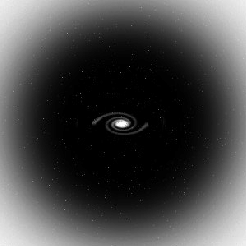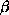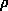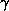Contra is a publicly available code that calculates the contraction of a dark matter halo in response to condensation of baryons in its center. The code is based on the modified contraction model of Gnedin et al. (2004).The following assumptions are made: The mass distribution of a dark matter halo is spherically symmetric and the velocity distribution is isotropic. The final baryon distribution does not need to be spherical. The code also calculates a line-of-sight velocity dispersion for a tracer population with a given density profile and velocity anisotropy (isotropic, constant, Osipkov-Merritt, or Mamon-Lokas model). Input options: There are two ways of specifying the initial dark matter distribution and the final baryon distribution. (i) Analytical distributions commonly used in astronomy: NFW, Exponential, Hernquist, Jaffe, Sersic, or generalized Spherical Sersic models. Tracer population can be one of the above profiles or a power law with a given slope. (ii) Numerical distributions in an optional input file. Units: G=1, Rvir=1, Mvir=1 for analytical distributions. For numerical input profiles, the unit of mass is arbitrary but the radii should be in units of the virial radius. All output quantities are in the input units, i.e. the velocity unit is (GMvir/Rvir)1/2. If you use this code for a publication, please acknowledge the original paper of the modified model: O. Y. Gnedin, A. V. Kravtsov, A. A. Klypin & D. Nagai, 2004, ApJ, 616, 16 "Response of dark matter halos to condensation of baryons: cosmological simulations and improved adiabatic contraction model"

 Download contra here NOTE: a new version of Contra with adjustable parameters A and w, referenced in    O. Y. Gnedin, D. Ceverino, N. Y. Gnedin, A. A. Klypin, A. V. Kravtsov, R. Levine, D. Nagai & G. Yepes, 2011, ApJ, submitted    "Halo Contraction Effect in Hydrodynamic Simulations of Galaxy Formation", is included in this distribution. The User Guide will be updated shortly and will explain the usage.

Contra User Guide (PDF)

Install:   Untar the distribution and type make. It will create an executable contra.

Command line arguments:

contra MAC DM BAR TRACE ANIS c serdm fb rb serb ra [in_file]

MAC (model of adiabatic contraction) : 0 = standard model, 1 = modified model Gnedin et al. (2004), -1 = no contraction
DM (initial dark matter profile) : 1 = NFW, 2 = Navarro et al. (2004), 3 = truncated satellite (Kazantzidis et al. 2004)
BAR (final baryon profile) : 1 = Exponential disk, 2 = Hernquist model, 3 = Jaffe model, 4 = Sersic (same m as DM)
TRACE (tracer population) : 1 = Exponential, 2 = Hernquist, 3 = Jaffe, 4 = Sersic (same m as DM), <0 = slope of a power law
ANIS (type of anisotropy distribution) : 0 = isotropic, 1 = constant, 2 = Mamon-Lokas, 3 = Osipkov-Merritt
c = initial halo concentration parameter, Rvir/rs
serdm = index of a spherical Sersic profile for dark matter
fb = baryon fraction within the virial radius, Mb/Mvir
rb = final baryon scalelength, rb/Rvir
serb = index of a Sersic profile for baryons (if TRACE=6), otherwise baryon truncation radius
ra = radius of velocity anisotropy for tracer population, ra/Rvir (or, for ANIS=1)
in_file = (optional) file with numerical profiles for initial dm and final baryons
six input columns: ri, mbi, mhi, rbf, mbf,tr

Note: the calculation of the line-of-sight velocity dispersion is the most computationally intensive part. If the dispersion is not needed, set TRACE=0 to avoid its computation.

Output:

ri = initial grid of radiii = initial dark matter density at rif = final dark matter density at rii = initial logarithmic slope of dark matter density at rif = final logarithmic slope of dark matter density at rilos = line-of-sight velocity dispersion of the tracer population at ri